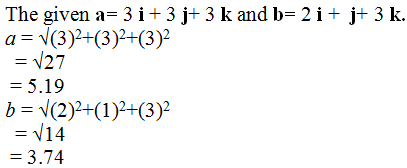×#### Thank you for registering.

One of our academic counsellors will contact you within 1 working day.

Click to Chat

1800-1023-196

+91-120-4616500

CART 0

• 0

MY CART (5)

Use Coupon: CART20 and get 20% off on all online Study Material

ITEM
DETAILS
MRP
DISCOUNT
FINAL PRICE
Total Price: Rs.

There are no items in this cart.
Continue Shopping
```
Use Eqs. 11-3 and 11 -5 to calculate the angle between the two vectors and

```
5 years agoNavjyot Kalra
654 Points
```By using equation (1),a . b = (3 i + 3 j+ 3 k). (2 i + j+ 3 k) = (3×2) + (3×1) +(3×3) =6+3+9 =18 …… (3)By using equation (2),a . b = (5.19) (3.74) cos ?So, cos ? = a . b/(5.19) (3.74)Φ = cos [a . b/(5.19) (3.74)] …… (4)To find out the angle between a and b, substitute 18 for a . b in the equation (4) we get,Φ = cos [a . b/(5.19) (3.74)] = cos [18/(5.19) (3.74)] = 22.0° …… (5)From equation (5) we observed that, the angle between a and b will be 22.0°.
```
5 years ago
Think You Can Provide A Better Answer ?

## Other Related Questions on Mechanics

View all Questions »### Course Features

• 101 Video Lectures
• Revision Notes
• Previous Year Papers
• Mind Map
• Study Planner
• NCERT Solutions
• Discussion Forum
• Test paper with Video Solution### Course Features

• 110 Video Lectures
• Revision Notes
• Test paper with Video Solution
• Mind Map
• Study Planner
• NCERT Solutions
• Discussion Forum
• Previous Year Exam Questions